# Sum three fractions

Work out the sum of 1/4, 1/5 and 3/10.

s =  0.75

### Step-by-step explanation: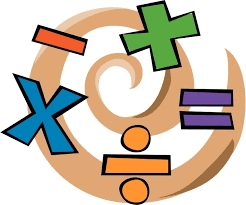Did you find an error or inaccuracy? Feel free to write us. Thank you!Tips to related online calculators
Need help to calculate sum, simplify or multiply fractions? Try our fraction calculator.

## Related math problems and questions:What is the sum of 2/3 and 3/10?Why does 1 3/4 + 2 9/10 equal 4.65? How do you solve this?
• Product of the sum and differenceCalculate the product of the sum and difference of numbers -7 and -2.
• Tartlets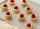After a special event, a caterer examined the leftovers on the serving table. She saw 10/11 of a tartlet with apples, 2/3 of a tartlet with strawberries, and 10/11 of a tartlet with raspberries. How many leftover tartlets did the caterer have?Add two mixed fractions: 2 4/6 + 1 3/6To three-eighths of one third, we add five quarters of one half and multiply the sum by four. How much will we get?
• FractionsSort fractions z1 = (6)/(11); z2 = (10)/(21); z3 = (19)/(22) by its size. Result write as three serial numbers 1,2,3.
• Product and sumWhat is the product of two fourths  and the sum of three halves and four?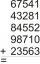Fill letters instead of digits so the indicated sum (equal letters represent equal digits). What number is hidden under the letter J? A A H A H O A H O J -------------------------- 4 3 2 1
• The sumThe sum of the first 10 members of the arithmetic sequence is 120. What will be the sum if the difference is reduced by 2?7 is added to the sum of 4/5 and 6/7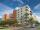The residential house has three entrances numbered even numbers, successive immediately behind. The sum of the two numbers on the outside entrances is 68. Calculate the middle of these three numbers.Sum of the two numbers is 15 1/6. If one of the numbers is 2 2/3, find the other number.Add this two mixed numbers: 1 5/6 + 2 2/11=What must be subtracted from the sum of 3/8 and 5/16 to get difference equal to the product of 5/8 and 3/16?If you travel to work 22 days and it takes 29.2 minutes, how many minutes will it take to travel to work and back?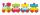The numbers 1,2,3,4,5,6,7,8 and 9 traveled by train. The train had three cars and each was carrying just three numbers. No. 1 rode in the first carriage, and in the last carriage was all odd numbers. The conductor calculated sum of the numbers in the firs